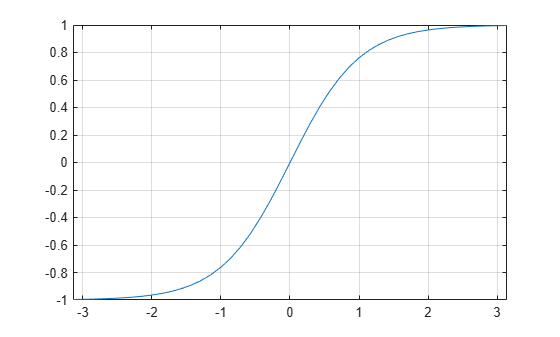# tanh

Symbolic hyperbolic tangent function

## Syntax

``tanh(X)``

## Description

example

````tanh(X)` returns the hyperbolic tangent function of `X`.```

## Examples

### Hyperbolic Tangent Function for Numeric and Symbolic Arguments

Depending on its arguments, `tanh` returns floating-point or exact symbolic results.

Compute the hyperbolic tangent function for these numbers. Because these numbers are not symbolic objects, `tanh` returns floating-point results.

`A = tanh([-2, -pi*i, pi*i/6, pi*i/3, 5*pi*i/7])`
```A = -0.9640 + 0.0000i 0.0000 + 0.0000i 0.0000 + 0.5774i... 0.0000 + 1.7321i 0.0000 - 1.2540i```

Compute the hyperbolic tangent function for the numbers converted to symbolic objects. For many symbolic (exact) numbers, `tanh` returns unresolved symbolic calls.

`symA = tanh(sym([-2, -pi*i, pi*i/6, pi*i/3, 5*pi*i/7]))`
```symA = [ -tanh(2), 0, (3^(1/2)*1i)/3, 3^(1/2)*1i, -tanh((pi*2i)/7)]```

Use `vpa` to approximate symbolic results with floating-point numbers:

`vpa(symA)`
```ans = [ -0.96402758007581688394641372410092,... 0,... 0.57735026918962576450914878050196i,... 1.7320508075688772935274463415059i,... -1.2539603376627038375709109783365i]```

### Plot Hyperbolic Tangent Function

Plot the hyperbolic tangent function on the interval from $-\pi$ to $\pi$.

```syms x fplot(tanh(x),[-pi pi]) grid on```### Handle Expressions Containing Hyperbolic Tangent Function

Many functions, such as `diff`, `int`, `taylor`, and `rewrite`, can handle expressions containing `tanh`.

Find the first and second derivatives of the hyperbolic tangent function:

```syms x diff(tanh(x), x) diff(tanh(x), x, x)```
```ans = 1 - tanh(x)^2 ans = 2*tanh(x)*(tanh(x)^2 - 1)```

Find the indefinite integral of the hyperbolic tangent function:

`int(tanh(x), x)`
```ans = log(cosh(x))```

Find the Taylor series expansion of `tanh(x)`:

`taylor(tanh(x), x)`
```ans = (2*x^5)/15 - x^3/3 + x```

Rewrite the hyperbolic tangent function in terms of the exponential function:

`rewrite(tanh(x), 'exp')`
```ans = (exp(2*x) - 1)/(exp(2*x) + 1)```

## Input Arguments

collapse all

Input, specified as a symbolic number, variable, expression, or function, or as a vector or matrix of symbolic numbers, variables, expressions, or functions.

## Version History

Introduced before R2006a